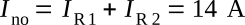Home   >   Textbooks   >   Basic Electronics   >   DC Network Analysis   >   Norton's Theorem   >

DC Network Analysis

# Norton's Theorem

Norton's theorem provides a second method of reducing complex circuitry to a simple equivalent circuit.

Norton's theorem is especially useful in analyzing circuits where only one particular resistor in the circuit (called the load) is subject to change. Example:Example circuit.

Norton's theorem states: Any linear electrical circuit can be replaced at terminals A and B by a simple parallel circuit consisting of an equivalent current source Ino and an equivalent resistance Rno.

The equivalent parallel circuit will provide the same current through a load as would the original circuit. The equivalent current Ino is the current that would flow through a short circuit between the two terminals (A and B) being considered in the original network. The equivalent resistance Rno is the resistance "seen" between the two terminals being considered in the original network when all voltage sources of the original circuit are replaced by a short circuit (wire) and all current sources are replaced by an open circuit (break).Norton equivalent circuit.

The figure below shows the application of Norton's theorem to a simple network. When Norton's theorem is applied, the current through RL of the figure below (view A) is the same as the current in RL of the figure below (view D).Transition of a network to resultant Norton-equivalent circuit.

In Norton's theorem, as in Thevenin's theorem, the equivalency is established for the chosen load terminals. Therefore, the equivalency applies only at the load.

Application of Norton's theorem is further illustrated in the following examples.

Example 1:
Find the Norton-equivalent circuit of the circuit shown in the figure below.Example circuit.

Solution:

Calculating the equivalent current InoShorted-terminal circuit used to calculate Ino.

Place a wire (short) between the points A and B (figure above), and use Ohm's law to calculate the currents through resistors R1 and R2:The current through the short between the points A and B is the sum of the currents IR1 and IR2:Calculating the equivalent resistance Rno

Replace the voltage sources V1 and V2 with a wire:Circuit used to calculate Rno.

The resistance between the points A and B is equal to R1 and R2 in parallel: 0.8 Ω. This is our equivalent resistance Rno in the equivalent circuit:Final equivalent circuit.

Example 2:
Find the Norton-equivalent circuit of the circuit shown in the figure below.Example circuit.

Solution:

Calculating the equivalent current InoShorted-terminal circuit used to calculate Ino.

Place a wire (short) between the points A and B (figure above), and use Ohm's law to calculate the currents through resistor R2:The current through the short between the points A and B is the sum of the currents IR1 and IR2:Calculating the equivalent resistance Rno

Replace the current source I1 with a break and the voltage source V2 with a wire:Circuit used to calculate Rno.

The resistance between the points A and B is equal to R2: 1 Ω. This is our equivalent resistance Rno in the equivalent circuit:Final equivalent circuit.